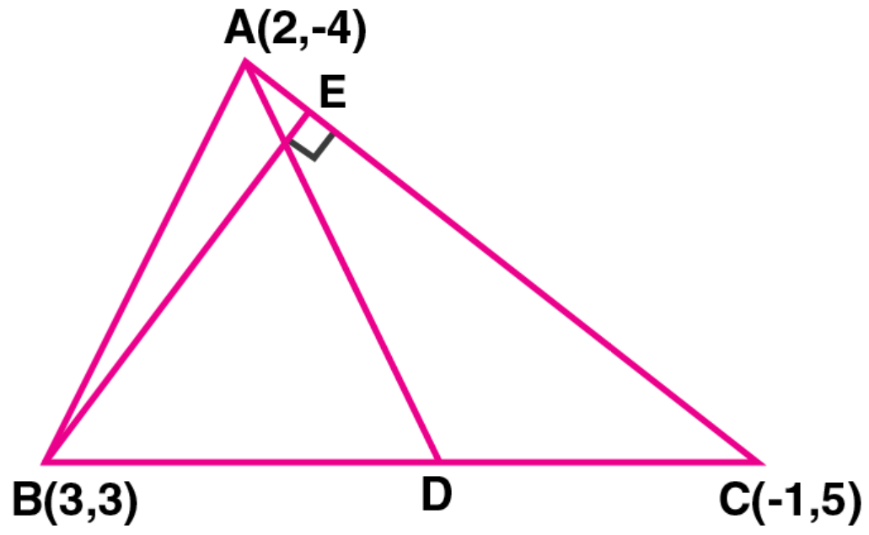Newbie

# A (2, – 4), B (3, 3) and C (– 1, 5) are the vertices of triangle ABC. Find the equation of: (i) the median of the triangle through A (ii) the altitude of the triangle through B.

• 0

This is the Important question of class 10 Based on Equation of a Straight Line Chapter of M.L Aggarwal book for ICSE BOARD.
Here vertices of a trianlge is given.
Find the equation of the median of the triangle through a point and the altitude of the triangle through a point.
This is the Question Number 33, Exercise 12.2 of M.L Aggarwal.

Share

1.Given, A (2, – 4), B (3, 3) and C (– 1, 5) are the vertices of triangle ABC

(i) D is the mid-point of BC

So, the co-ordinates of D will be

((3 – 1)/2, (3 + 5)/2) = (2/2, 8/2) = (1, 4)

Now,

The slope of AC (m1) = (5 + 4)/ (-1 – 2) = 9/-3 = -3

Let the slope of BE be m2

Then, m1 x m2 = -1

-3 x m2 = -1

m2 = 1/3

so, the equation of BE will be

y – 3 = 1/3 (x – 3)

3y – 9 = x – 3

x – 3y + 6 = 0

Thus, the required line equation is x – 3y + 6 = 0.

• -3